Ch 7. Incompressible and Inviscid Flow Multimedia Engineering Fluids Bernoulli'sEquation FlowMeasurements Superpositionof Flows Flow aroundCylinder
 Chapter 1. Basics 2. Fluid Statics 3. Kinematics 4. Laws (Integral) 5. Laws (Diff.) 6. Modeling/Similitude 7. Inviscid 8. Viscous 9. External Flow 10. Open-Channel Appendix Basic Math Units Basic Equations Water/Air Tables Sections Search eBooks Dynamics Fluids Math Mechanics Statics Thermodynamics Author(s): Chean Chin Ngo Kurt Gramoll ©Kurt GramollFLUID MECHANICS - CASE STUDY SOLUTION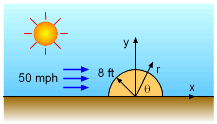Problem Description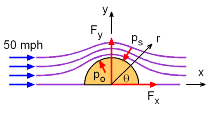Drag and Lift Based on the analysis of potential flow past a cylinder discussed in the theory section, the pressure on the cylinder is given by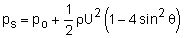The drag and lift can then be determined by integrating the pressure over the cylinder. Instead of integrating over the whole cylinder as was done in the theory section, the integration is taken over a half-cylinder (i.e., from 0 to π). However, the above equation is for only outside pressure. Inside pressure, po, must also be included. First, the total drag acting on the hut is,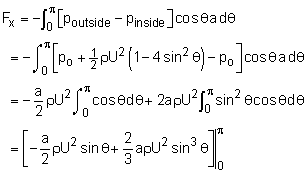Fx = 0 - 0 = 0 As expected, there is no drag on the hut since the solutions are obtained based on the assumption of inviscid flow.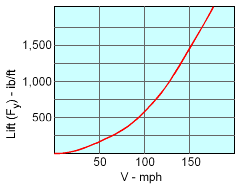Plot of Lift Versus Upstream Velocity Similarly, the lift is calculated as,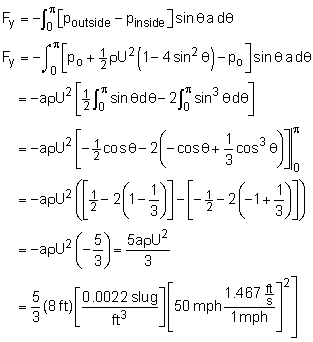Fy = 157.8 lb/ft The calculated lift for this particular problem is acting upward, as expected. While 158 lb/ft is not too much lift, if the velocity increases to 150 mph, the lift will be 1,420 lb/ft. This is almost 10 times the lift force. This is one reason why roofs are ripped off during high wind storms.

Practice Homework and Test problems now available in the 'Eng Fluids' mobile app
Includes over 250 problems with complete detailed solutions.
Available now at the Google Play Store and Apple App Store.